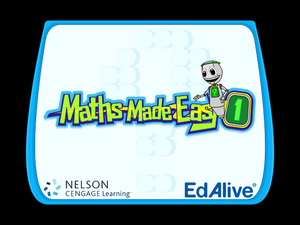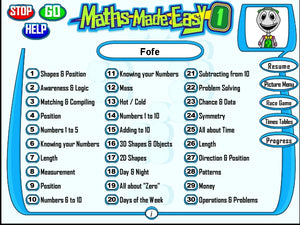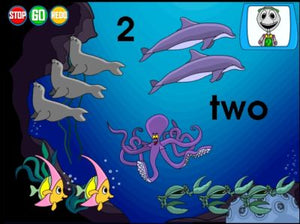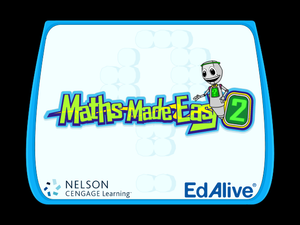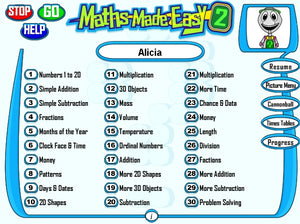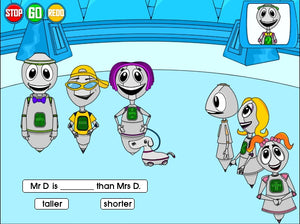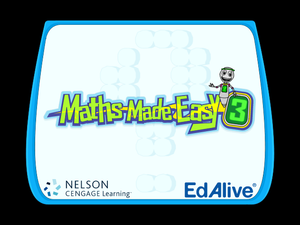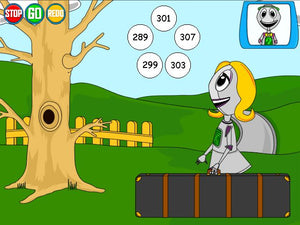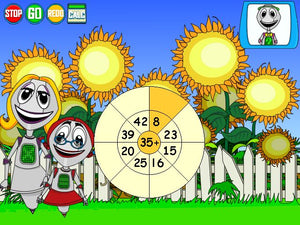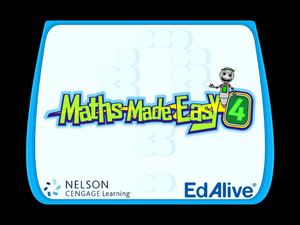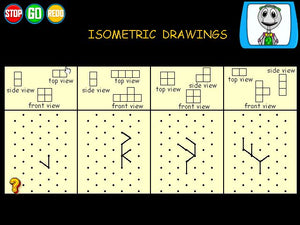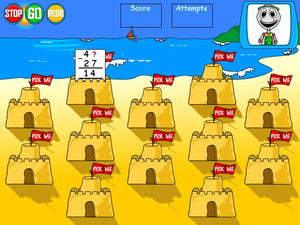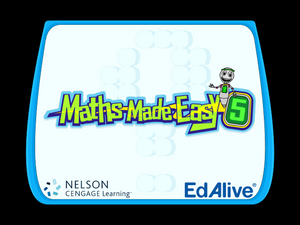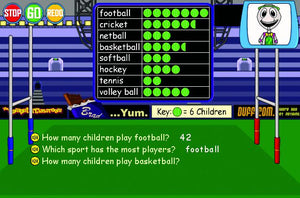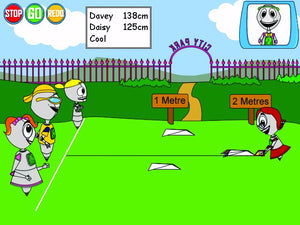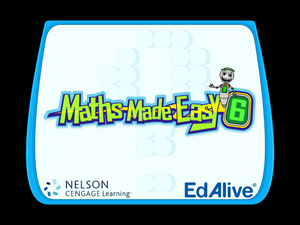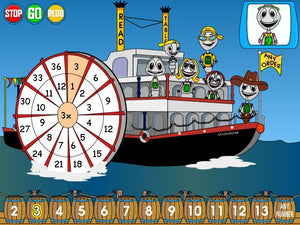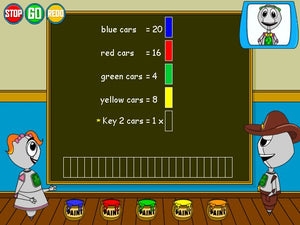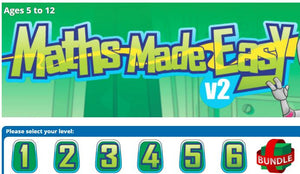Edalive

Regular price \$49.95 Sale

## Maths Made Easy v2: Series Overview ages 5-12

### This version includes unlimited download availability for peace of mind. If you have to restore your computer or get a new one, you can re-download it at any time using the link we send you.

• Uses lively images, animations and sounds for a truly interactive experience
• Includes fun animated lessons and demonstrations
• Features a cool range of engaging single/multi-player games!
• Caters for all learning styles with an exceptionally diverse range of activities
• Uses positive reinforcement and appropriate feedback
• Contains printable progress reports, lessons and certificates of achievement

Why you will love Maths Made Easy:

• Provides a solution-oriented approach to learning
• Features a comprehensive range of progressive, age-appropriate lessons and activities
• Monitors the progress of up to 40 users to allow targeting of individual needs for extension and revision
• Contains printable progress reports, lessons and certificates of achievement
• Includes clear, careful instructions and explanations
• Provides opportunities to learn and practise
• All titles work together as an integrated, progressive learning package

The educational quality of Maths Made Easy:

• Designed by experienced teachers
• Follows recognised principles in developmental educational psychology
• Based on educational progressions that underpin the curricula for all Australian states
• Focuses on the process as well as the product, helping students think carefully and logically
• Uses British English spelling conventions and SI units of measurement (metres, kilograms, kilometres, etc.)

### Educational Content

Level 1:

• Measurement
• hot/cold; day/night
• Days of the week
• Time
• Length
• Direction
• Position
• 2D shapes
• Awareness & logic
• Matching & compiling
• Symmetry
• 3D objects
• Mass
• Numbers 1 to 10
• Subtracting from 10
• Operations & problems
• Money
• Problem solving
• Chance & data
• And patterns

Level 2:

• Months of the year
• Clock face & time
• Days & dates
• Temperature
• Length
• 2D shapes
• Patterns
• 3D objects
• Mass
• Volume
• Numbers 1 to 20
• Ordinal numbers
• Subtraction
• Multiplication
• Division
• Fractions
• Money
• Chance & data
• Problem solving

Level 3:

• Temperature
• Time
• 2D shapes
• Angles
• Length
• Area
• 3D objects
• Volume
• Mass
• Numeration
• Subtraction
• Multiplication
• Division
• Fractions
• Money
• Data & graphs
• Using calculators
• Working mathematically
• Problem solving

Level 4:

• Temperature
• Time
• 2D shapes & angles
• Position
• Length
• Area
• 3D objects
• Volume
• Mass
• Numeration
• Subtraction
• Multiplication
• Division
• Fractions
• Data & graphs
• Working Mathematically
• Problem Solving

Level 5:

• Temperature
• Time
• Length
• Area
• Position
• 2D shapes & angles
• 3D objects
• Volume
• Mass
• Numeration
• Subtraction
• Multiplication
• Division
• Fractions
• Money
• Data & graphs
• Working mathematically
• Problem solving

Level 6:

• Temperature
• Time
• 2D shapes & angles
• Position
• Length
• Area
• 3D objects
• Volume
• Mass
• Numeration
• Subtraction
• Multiplication
• Division
• Fractions & decimals
• Data & graphs
• Working Mathematically
• Problem Solving